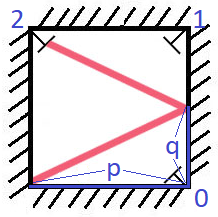858. Mirror Reflection

There is a special square room with mirrors on each of the four walls.  Except for the southwest corner, there are receptors on each of the remaining corners, numbered `0`, `1`, and `2`.

The square room has walls of length `p`, and a laser ray from the southwest corner first meets the east wall at a distance `q` from the `0`th receptor.

Return the number of the receptor that the ray meets first.  (It is guaranteed that the ray will meet a receptor eventually.)

Example 1:

```Input: p = 2, q = 1
Output: 2
Explanation: The ray meets receptor 2 the first time it gets reflected back to the left wall.```

Note:

1. `1 <= p <= 1000`
2. `0 <= q <= p`

858. Mirror Reflection
``````struct Solution;

impl Solution {
fn mirror_reflection(mut p: i32, mut q: i32) -> i32 {
while p % 2 == 0 && q % 2 == 0 {
p /= 2;
q /= 2;
}
if p % 2 == 0 {
return 2;
}
if q % 2 == 0 {
return 0;
}
1
}
}

#[test]
fn test() {
let p = 2;
let q = 1;
let res = 2;
assert_eq!(Solution::mirror_reflection(p, q), res);
}
``````